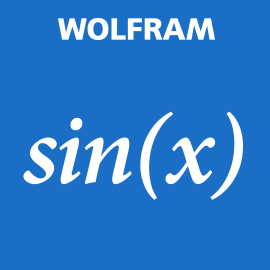\$1.99
Overview System Requirements Related

## Description

Taking precalculus? Then you need the Wolfram Precalculus Course Assistant. This definitive app for precalculus—from the world leader in math software—will help you work through your homework problems, ace your tests, and learn calculus concepts. Forget canned examples! The Wolfram Precalculus Course Assistant solves your specific precalculus problems on the fly, including solving equations, vector arithmetic, statistics, and much more. This app covers the following topics applicable to precalculus and trigonometry: - Evaluate any numeric expression or substitute a value for a variable - Solve a single equation or a system of equations - Plot functions on the x-y plane or draw a parametric or polar plot - Determine the sine, cosine, and tangent of a specific angle in a right triangle - Simplify, expand, or factor trigonometric functions - Find the partial fraction decomposition of an expression - Calculate the dot product, cross product, and magnitude of two vectors - Identify the mean, median, mode, and standard deviation of a set of data - Calculate permutations and combinations The Wolfram Precalculus Course Assistant is powered by the Wolfram|Alpha computational knowledge engine and is created by Wolfram Research, makers of Mathematica—the world's leading software system for mathematical research and education. The Wolfram Precalculus Course Assistant draws on the computational power of Wolfram|Alpha's supercomputers over a 3G, 4G, or Wi-Fi connection.

## People also like

Wolfram Group LLC

#### Developed by

Wolfram Group LLC

12/15/2014

1.21 MB

For all ages

#### This app can

Access your Internet connection and act as a server.
Access your home or work networks

#### Installation

Get this app while signed in to your Microsoft account and install on up to ten Windows 10 devices.

#### Language supported

English (United States)